﻿ 基于中心性的路侧单元部署算法设计

# 基于中心性的路侧单元部署算法设计The Algorithm Design for the Deployment of RSUs Based on Centrality

Abstract: This paper studies the deployment of road side units with limited cost in suburban scenarios. We introduce the concept of centrality and analyze the deployment of RSUs in the case of Degree-Centricity and Betweeness-Centricity, respectively. In the process of analyzing and modeling, the time coverage of vehicles travelling at a uniform speed is considered. Simulation results verify the rationality of the two kinds of deployment scheme and show that the time coverage of Degree-centric deployment is better than that of random deployment, when the time coverage of Betweeness-centric deployment is similar to that of random deployment.

1. 引言

2. 网络模型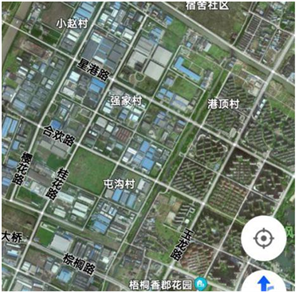Figure 1. Satellite map of a place in Zhong Lou area of Changzhou City, Jiangsu Province

3. 中心性概念

1) 度中心性(Degree Centrality, DC)：在无向图

${C}_{D}\left({N}_{i}\right)=\underset{j=1}{\overset{g}{\sum }}{x}_{ij}\left(i\ne j\right)$ (1)

${{C}^{\prime }}_{D}\left({N}_{i}\right)=\frac{{C}_{D}\left({N}_{i}\right)}{g-1}$ (2)

2) 中介中心性(Betweeness Centrality, BC)：中介指在不同事物或同一事物内部对立的两极之间起居间联系作用的环节。中介中心性就是一个节点的联系作用的衡量标准,其计算公式：

${C}_{B}\left(v\right)=\underset{s\ne v\ne t\in V}{\sum }\frac{{\sigma }_{st}\left(v\right)}{{\sigma }_{st}}$ (3)

4. 问题描述Figure 2. A simplified map of suburban road

5. 基于中心性的路侧单元部署算法设计

$S\left(i,a\right)=\mathrm{max}\left(S\left(i+1,a\right),S\left(i+1,a-p\left(i\right)\right)+c\left(i\right)\right),\text{\hspace{0.17em}}\text{\hspace{0.17em}}a\ge p\left(i\right);$ (6)

$S\left(i,a\right)=S\left(i+1,a\right)\text{,}\text{\hspace{0.17em}}\text{\hspace{0.17em}}a (7)

$S\left(N,a\right)=c\left(N\right)\text{,}\text{\hspace{0.17em}}\text{\hspace{0.17em}}a\ge p\left(N\right);$ (8)

$S\left(N,a\right)=0,\text{\hspace{0.17em}}\text{\hspace{0.17em}}a (9)

$a=B$$i=1,2,3,\cdots ,N$ 时就得到了最优解。即 $S\left(1,B\right)$ 就是总中心性最大的值，此时可以从候选点1开始回溯，a从B开始降低，以2500作为步进值，当 $S\left(i,a\right)=S\left(i+1,a\right)$ 说明第i个候选点没有部署路侧单元，反之则部署，部署后将a减去p(i)继续运行。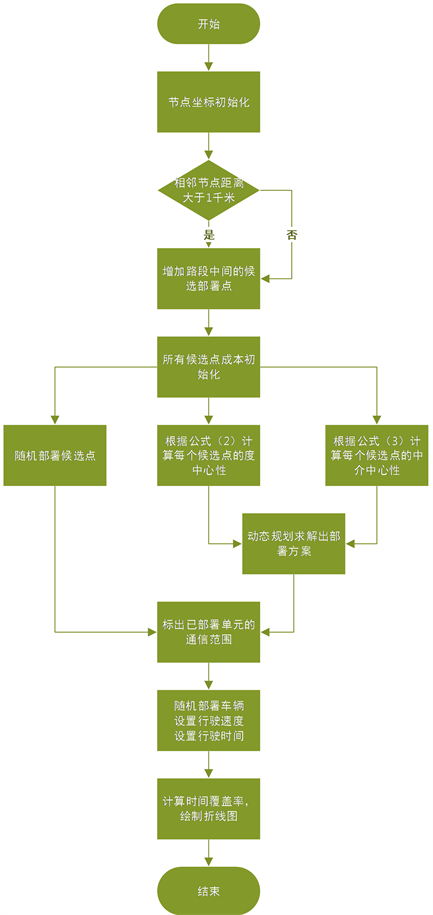Figure 3. Algorithm flowchart

6. 数值结果分析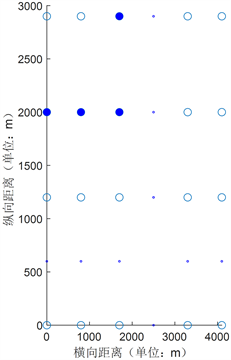Figure 4. Intersection and candidate point simulation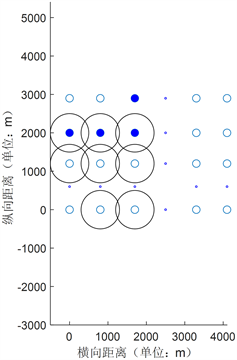Figure 5. B is 150,000 yuan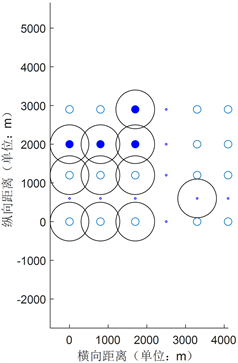Figure 6. B is 200,000 yuan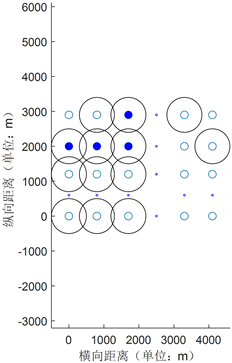Figure 7. B is 250,000 yuan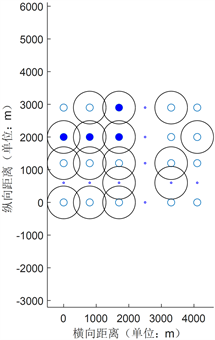Figure 8. B is 300,000 yuan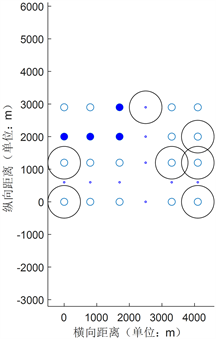Figure 9. B is 150,000 yuanFigure 10. B is 200,000 yuan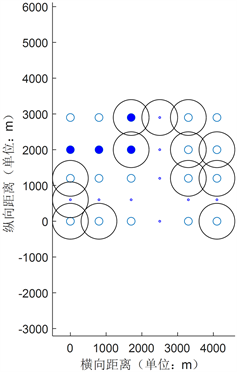Figure 11. B is 250,000 yuan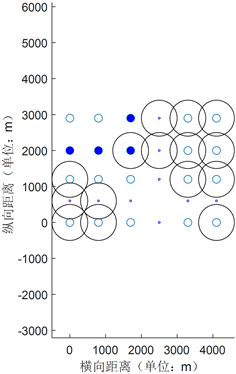Figure 12. B is 300,000 yuan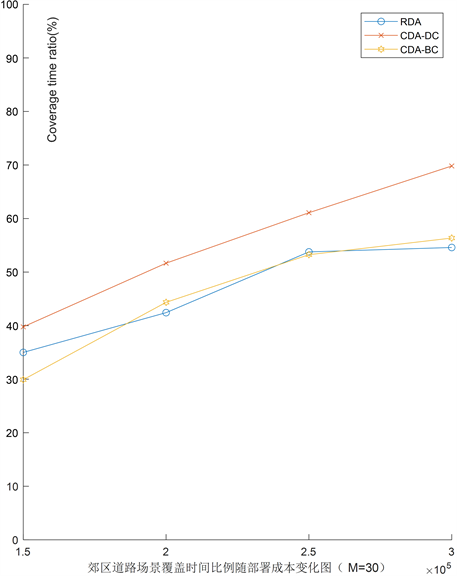Figure 13. The relationship between time coverage and cost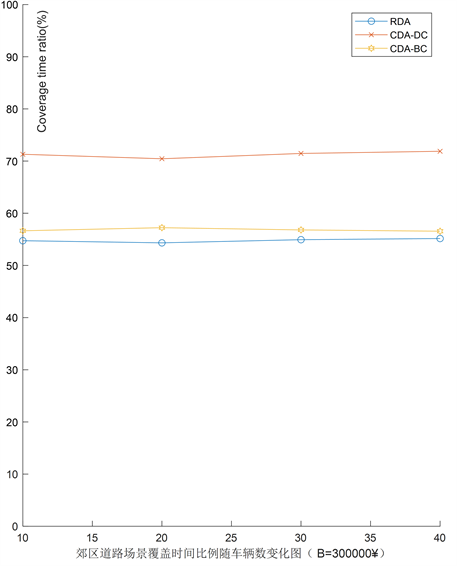Figure 14. The relationship between time coverage and the number of vehicles

7. 总结

NOTES

*通讯作者。

 赵春明. 私人交通、城市扩张与雾霾污染——基于65个大中城市面板数据的实证分析[J]. 财贸研究, 2020(10): 20-29.

 喻潇. 2010-2015年北京市海淀区居民伤害死亡特征分析[J]. 疾病监测, 2019, 34(2): 166-170.

 崔洪军. 基于节能减排的高速公路收费站处拥挤车流控制技术[J]. 中国公路学报, 2015, 28(5): 125-129.

 孟晓彤. 基于实时路况的兰州市城关区交通拥堵时空特征及对策探究[D]: [硕士学位论文]. 兰州: 兰州大学, 2018.

 苏明. 汽车节油辅助驾驶系统研究[D]: [硕士学位论文]. 长春: 吉林大学, 2015.

 刘凯利. 个人驾驶行为的评估与分析研究[D]: [硕士学位论文]. 北京: 北方工业大学, 2017.

 魏涛. 车联网环境下汽车节能驾驶行为与速度优化方法研究[D]: [硕士学位论文]. 西安: 长安大学, 2019.

 李志龙. 车联网中路侧单元部署策略研究[D]: [硕士学位论文]. 上海: 上海交通大学, 2019.

 Future论坛. 车车通信技术白皮书[Z]. 20171110.

 朱涵. 车联网路侧单元部署算法研究[D]: [硕士学位论文]. 大连: 大连理工大学, 2016.

 吴青文. 车载自组织网中路边单元部署问题研究[D]: [硕士学位论文]. 兰州: 西北师范大学, 2019.

 王振宇. 车载自组织网路路侧单元部署方法研究[D]: [硕士学位论文]. 南京: 东南大学, 2017.

 范敏. 基于矩阵分解技术的社会网络中节点中心性算法的研究[D]: [硕士学位论文]. 济南: 山东大学, 2020.

 吴吟. 互联网信息传播中的事件网络关键节点研究——一项基于网络拓扑学的实证研究[J]. 中国广播电视学刊, 2020(6): 37-42.

 蓝雯飞. 背包问题的动态规划改进算法[J]. 中南民族大学学报(自然科学版), 2016, 35(4): 101-105.

Top